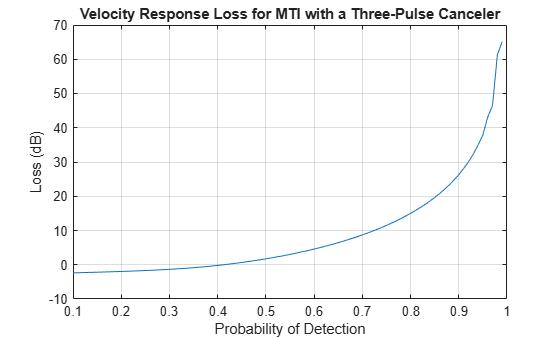# mtiloss

Losses due to moving target indicator (MTI) processing

## Syntax

``[LI,LV] = mtiloss(PD,PFA,N)``
``[LI,LV] = mtiloss(PD,PFA,N,M)``
``[LI,LV] = mtiloss(PD,PFA,N,M,SW)``
``[LI,LV] = mtiloss(___,Name,Value)``
``[___,LBP] = mtiloss(___)``

## Description

````[LI,LV] = mtiloss(PD,PFA,N)` computes integration loss, `LI`, and velocity response loss, `LV`, due to MTI processing with a two-pulse (first-order) canceller given the probability of detection, `PD`, probability of false alarm, `PFA`, and the number of received pulses available at the MTI input, `N`.The function computes the loss assuming you are using a square-law detector and a nonfluctuating target. ```

example

````[LI,LV] = mtiloss(PD,PFA,N,M)` computes losses due to MTI processing with an `M`-pulse canceler. ```

example

````[LI,LV] = mtiloss(PD,PFA,N,M,SW)` computes MTI losses for radar echoes received from a chi-squared distributed target specified using the Swerling case number, `SW`. ```
````[LI,LV] = mtiloss(___,Name,Value)` computes MTI losses using one or more name-value arguments. For example, ```[LI,LV] = mtiloss(0.64,1e-12,8,'Method','Batch')``` calculates `LI` and `LV` for MTI with batch processing. Specify the name-value arguments after any of the input arguments from the previous syntax.```

example

````[___,LBP] = mtiloss(___)` computes the blind phase loss `LBP` only when you set `IsQuadrature` name-value argument to `false`. ```

## Examples

collapse all

Calculate the velocity response loss for an MTI processing with a three-pulse canceler, with the probability of false alarm of `1e-6` and `24 `pulses received from a nonfluctuating target.

```PFA = 1e-6; N = 24; M = 3; PD = 0.1:0.01:0.99; [~,LV] = mtiloss(PD,PFA,N,M);```

Plot the velocity response loss.

```plot(PD,LV) xlabel('Probability of Detection') ylabel('Loss (dB)') title('Velocity Response Loss for MTI with a Three-Pulse Canceler') grid on```Compute the noise correlation loss for MTI processing with a three-pulse canceler. Assume that the desired probability of detection is `0.9`, the probability of false alarm is `1e-6`, and `24` pulses are received from a Swerling 1 target.

```PD = 0.9; PFA = 1e-6; N = 24; M = 3; LI = mtiloss(PD,PFA,N,M,'Swerling1')```
```LI = 2.0811 ```

Compute the blind phase loss for an MTI with a two-pulse canceler with the desired probability of detection of `0.95`, the probability of false alarm of `1e-8`, and `10` pulses received from a nonfluctuating target.

```PD = 0.95; PFA = 1e-8; N = 10; [~,~,LBP] = mtiloss(PD,PFA,N,'IsQuadrature',false)```
```LBP = 2.3881 ```

## Input Arguments

collapse all

Probability of detection in the range [`0.1,0.999999`], specified as a positive scalar or as a length-J vector with each element in the range [`0.1,0.999999`] .

Probability of false alarm, specified as a positive scalar in the range [`1e-15,1e-3`] or as a length-K vector with each element in the range [`1e-15,1e-3`] .

Number of received pulses available at the input of the MTI, specified as a positive integer equal to or greater than `2`.

Number of pulses in an M-pulse MTI canceler, specified as a positive integer in the range `[2,15]`. The `M`-pulse canceler is constructed using cascading `M`-1 two-pulse cancellers.

Swerling case number, specified as one of these

• `'Swerling0'`

• `'Swerling1'`

• `'Swerling2'`

• `'Swerling3'`

• `'Swerling4'`

• `'Swerling5'`

.

### Name-Value Pair Arguments

Specify optional comma-separated pairs of `Name,Value` arguments. `Name` is the argument name and `Value` is the corresponding value. `Name` must appear inside quotes. You can specify several name and value pair arguments in any order as `Name1,Value1,...,NameN,ValueN`.

Example: ```[LI,LV] = mtiloss(0.7,1e-8,10,'Method','Batch')```

Pulse processing method, specified as a character vector or string scalar.

• If you set `'Method'` to `'Sequential'`, the received pulses are processed sequentially resulting in `N`-`M` pulses at the output of the pulse canceler.

• If you set `'Method'` to `'Batch'`, the `N` received pulses are divided into `N`/(`M`+1) batches, which are processed separately resulting in `N`/(`M`+1) pulses at the output of the MTI.

Example: ```[LI,LV] = mtiloss(0.7,1e-9, 8,'Method','Batch')```

Quadrature-channel (vector) or single-channel MTI processing, specified as a logical value.

• If you set `'IsQuadrature'` to `true`, the MTI processing has two parallel cancelers for the I and Q components. By default, the function sets `'IsQuadrature'` to `true` and the blind phase loss output is zero.

• If you set `'IsQuadrature'` to `false`, only the I or the Q channel is used for MTI resulting in blind phase loss `LBP`.

Example: ```[LI,LV,LBP] = mtiloss(0.9,1e-8,10,'IsQuadrature',false)```

## Output Arguments

collapse all

Integration loss due to correlation in the noise samples at the output of the MTI filter, returned as a J-by-K matrix in dB with rows corresponding to the values in `PD` and columns to the values in `PFA`.

Velocity response loss due to target velocity lying near the null of the MTI pulse canceler, returned as a J-by-K matrix in dB with rows corresponding to the values in `PD` and columns to the values in `PFA`.

Blind phase loss, returned as a J-by-K matrix in dB with rows corresponding to the values in `PD` and columns to the values in `PFA`. `LBP` is computed only when you set the value of the `'IsQuadrature'` argument to `false`.